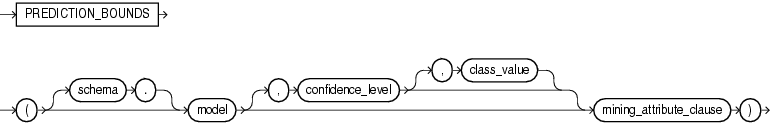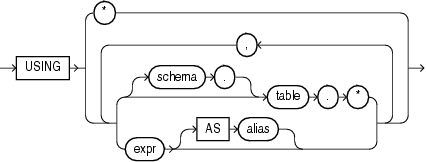## PREDICTION_BOUNDS

SyntaxDescription of the illustration prediction_bounds.eps

mining_attribute_clause::=Description of the illustration mining_attribute_clause.eps

Purpose

`PREDICTION_BOUNDS` applies a Generalized Linear Model (GLM) to predict a class or a value for each row in the selection. The function returns the upper and lower bounds of each prediction in a varray of objects with fields `UPPER` and `LOWER`.

GLM can perform either regression or binary classification:

• The bounds for regression refer to the predicted target value. The data type of `UPPER` and `LOWER` is the data type of the target.

• The bounds for binary classification refer to the probability of either the predicted target class or the specified `class_value`. The data type of `UPPER` and `LOWER` is `BINARY_DOUBLE`.

If the model was built using ridge regression, or if the covariance matrix is found to be singular during the build, then `PREDICTION_BOUNDS` returns `NULL` for both bounds.

`confidence_level` is a number in the range (0,1). The default value is 0.95. You can specify `class_value` while leaving `confidence_level` at its default by specifying `NULL` for `confidence_level`.

The syntax of the `PREDICTION_BOUNDS` function can use an optional `GROUPING` hint when scoring a partitioned model. See GROUPING Hint.

mining_attribute_clause

`mining_attribute_clause` identifies the column attributes to use as predictors for scoring. This clause behaves as described for the `PREDICTION` function. (Note that the reference to analytic syntax does not apply.) See .

Note:

The following example is excerpted from the Data Mining sample programs. For more information about the sample programs, see Appendix A in Oracle Data Mining User's Guide.

Example

The following example returns the distribution of customers whose ages are predicted with 98% confidence to be greater than 24 and less than 46.

```SELECT count(cust_id) cust_count, cust_marital_status
FROM (SELECT cust_id, cust_marital_status
FROM mining_data_apply_v
WHERE PREDICTION_BOUNDS(glmr_sh_regr_sample,0.98 USING *).LOWER > 24 AND
PREDICTION_BOUNDS(glmr_sh_regr_sample,0.98 USING *).UPPER < 46)
GROUP BY cust_marital_status;

CUST_COUNT CUST_MARITAL_STATUS
-------------- --------------------
46 NeverM
7 Mabsent
5 Separ.
35 Divorc.
72 Married```